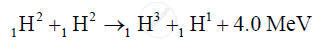Share

# Write One Balanced Equation To Show Nuclear Fusion - ISC (Arts) Class 12 - Physics (Theory)

ConceptNuclear Energy Nuclear Fusion – Energy Generation in Stars

#### Question

Write one balanced equation to show Nuclear fusion

#### Solution

Nuclear fusion :

The fusion of two nuclei of heavy hydrogen or deuterium ("_1H^2), the following is possibleIs there an error in this question or solution?

#### Video TutorialsVIEW ALL 

Solution Write One Balanced Equation To Show Nuclear Fusion Concept: Nuclear Energy - Nuclear Fusion – Energy Generation in Stars.
S